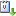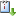Usted está aquí: On a priori bounds for positive solutions of subcritical elliptic problems

# On a priori bounds for positive solutions of subcritical elliptic problems

Ponente: Rosa Pardo
Tipo de Evento: Investigación
Cuándo 12/02/2020 de 12:00 a 13:00 Salón de seminarios Graciela SalicrupvCaliCal

Abstract:

We provide a priori bounds for positive solutions of $$p$$-Laplacian equations

$$-\Delta_p u =f(u)\quad \text{in}\ \Omega, \qquad u= 0\quad \text{on}\ \partial\Omega,$$

in a bounded domain, with a subcritical nonlinearity of the form

$$f(u)=u^{p^*-1}/[\ln (e+u)]^\alpha,$$

with $$p^*= Np/(N-p)$$ the critical Sobolev exponent and $$\alpha >p/(N-p)$$; see [1,2].

We extend our results to Hamiltonian elliptic systems

$$-\Delta u=f(v), \ -\Delta v=g(u)\ \text{in}\ \Omega, \ u=v=0\ \text{on}\ \partial\Omega,$$

where

$$f(v)=v^{p-1}/[\ln (e+v)]^\alpha,\quad g(u) =u^{q-1}/[\ln (e+u)]^\beta,$$

with $$\alpha, \beta> 2/(N-2)$$, and $$(p,q)$$ on the critical hyperbola $$\frac{1}{p} +\frac{1}{q} = \frac{N-2}{N}$$; see .

References:

 A. Castro and R. Pardo: A priori bounds for positive solutions of subcritical elliptic equations. Rev. Mat. Complut. 28 (2015), 715–731.

 L. Damascelli and R. Pardo: A priori estimates for some elliptic equations involving the p-Laplacian. Nonlinear Anal. 41 (2018), 475 – 496.

 N. Mavinga and R. Pardo: A priori bounds and existence of positive solutions for subcritical semilinear elliptic systems. J. Math. Anal. Appl. 449 (2017), 1172–1188.